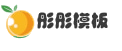# DEDECMS批量导入excel数据到后台文章系统的开发教程

2021-07-28 0 7,171

1）开发前的准备：2) 开发插入数据代码：

 ```header("Content-type:text/html;charset=utf8"); require_once('/../dedecms/include/common.inc.php'); error_reporting(E_ALL); set_time_limit(0); date_default_timezone_set('Europe/London'); /** Include path **/ set_include_path(get_include_path() . PATH_SEPARATOR . '../../../Classes/'); /** PHPExcel_IOFactory */ include 'PHPExcel/IOFactory.php'; if(!empty(\$_GET)){ \$typeid = \$_GET['typeid']; \$dopost = \$_GET['do']; if(\$dopost == "exdata"){ if(!empty(\$_GET['n'])){ \$inputFileName = './'.\$_GET['n'].'.xlsx'; \$objPHPExcel = PHPExcel_IOFactory::load(\$inputFileName); \$sheetData = \$objPHPExcel->getActiveSheet()->toArray(null,true,true,true); \$rowarr=array(); \$dsql->Execute('all',"select bio2,bio1 from `dede_addonshop`"); while (\$rowall = \$dsql->GetArray('all')) { \$rowarr[]=\$rowall; } foreach (\$sheetData as \$v) { foreach (\$rowarr as \$vt) { if(in_array(\$v['B'],\$vt)&&\$v['A']==\$vt['bio1']){ echo "货号为：".\$v['B']." 厂商为：".\$v['A']." 的数据已在表中，请在excel文件里面删除这一条后再添加！"; exit; } } } //附加表插入数据前处理 \$row = \$dsql->GetOne("select aid,bio2 from `dede_addonshop` order by aid desc"); if(!empty(\$row)){ \$aid = \$row['aid']; \$bio2 = \$row['bio2']; }else{ \$aid = 0; \$bio2 = ''; } //处理重复问题 if(\$bio2==\$sheetData[count(\$sheetData)]['B']){ ShowMsg("不能重复添加内容",'javascript:;'); exit; } //主表插入数据前处理 \$arcrow = \$dsql->GetOne("select id from `dede_archives` order by id desc"); if(!empty(\$arcrow)){ \$arcid = \$arcrow['id']; }else{ \$arcid = 0; } //微表插入数据前处理 \$tinyrow = \$dsql->GetOne("select id from `dede_arctiny` order by id desc"); if(!empty(\$tinyrow)){ \$tinyid = \$tinyrow['id']; }else{ \$tinyid = 0; } //找出最大的id \$id = max(\$aid,\$arcid,\$tinyid); \$alphalpha = array('A','B','C','D','E','F','G','H','I','J','K','L','M','N','O','P','Q','R','S','T','U','V','W','X','Y','Z','AA','AB','AC','AD','AE','AF','AG','AH','AI','AJ','AK','AL','AM','AN','AO','AP','AQ','AR','AS','AT','AU','AV','AW','AX','AY','AZ'); //字段数量 \$fieldnum = count(\$sheetData); \$fields = \$fieldvalue = ''; for (\$i=0; \$i < \$fieldnum; \$i++) { \$fields .= \$sheetData[\$alphalpha[\$i]]. ','; } \$fields = substr(\$fields, 0,-1); //遍历数组 foreach (\$sheetData as \$value) { \$pubdate = GetMkTime(GetDateTimeMk(time())); \$click = mt_rand(50, 200); if(\$value['A']=='bio1'|\$value['A']=='厂商'){ continue; } \$id = \$id+1; //获取字段值\$value['A']; for (\$i=0; \$i < \$fieldnum; \$i++) { \$fieldvalue .= " ,'".\$value[\$alphalpha[\$i]]."' "; } //标题 \$C = trim(\$value['C']); //保存到主表 \$senddate = time(); \$arcquery = "INSERT INTO `dede_archives`(id,typeid,title,mid,channel,pubdate,senddate,click,ismake)VALUES ('\$id','\$typeid','\$C','1','6','\$pubdate','\$senddate','\$click','-1');"; \$dsql->ExecuteNoneQuery(\$arcquery); //保存到附近加表 \$query = "INSERT INTO `dede_addonshop`(aid,typeid,\$fields) VALUES ('\$id','\$typeid'{\$fieldvalue});"; \$dsql->ExecuteNoneQuery(\$query); \$fieldvalue = ''; //保存到微表 \$tinyquery = "INSERT INTO `dede_arctiny`(id,typeid,channel,mid,senddate)VALUES ('\$id','\$typeid','6','1','\$senddate');"; \$dsql->ExecuteNoneQuery(\$tinyquery); } \$num = count(\$sheetData)-2; ShowMsg("恭喜，成功插入 ".\$num." 条数据！",'javascript:;'); } ```• 模板团承诺演示效果图均和网站95%一样，并且我们的模板和数据都没有留后门，请放心使用。查看详情
• 因为模板具有可复制性和可传播性，模板一旦发货，非质量问题，不支持退换；所以务必请您一定看清楚后再购买。查看详情

### 相关文章4个月前 2,8034个月前 2,8914个月前 3,7284个月前 3,482

• 0 +

访问总数

• 0 +

会员总数

• 0 +

资源总数

• 0 +

今日发布

• 0 +

本周发布

• 0 +

运行天数